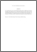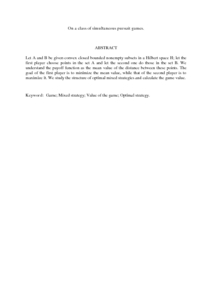Simple Search:

# On a class of simultaneous pursuit games.

## Citation

Ibragimov, Gafurjan I. and Satimov, Numan (2012) On a class of simultaneous pursuit games. Russian Mathematics , 56 (5). pp. 39-46. ISSN 1066-369X

## Abstract / Synopsis

Let A and B be given convex closed bounded nonempty subsets in a Hilbert space H; let the first player choose points in the set A and let the second one do those in the set B. We understand the payoff function as the mean value of the distance between these points. The goal of the first player is to minimize the mean value, while that of the second player is to maximize it. We study the structure of optimal mixed strategies and calculate the game value.Preview
PDF (Abstract)
On a class of simultaneous pursuit games.pdfView Item